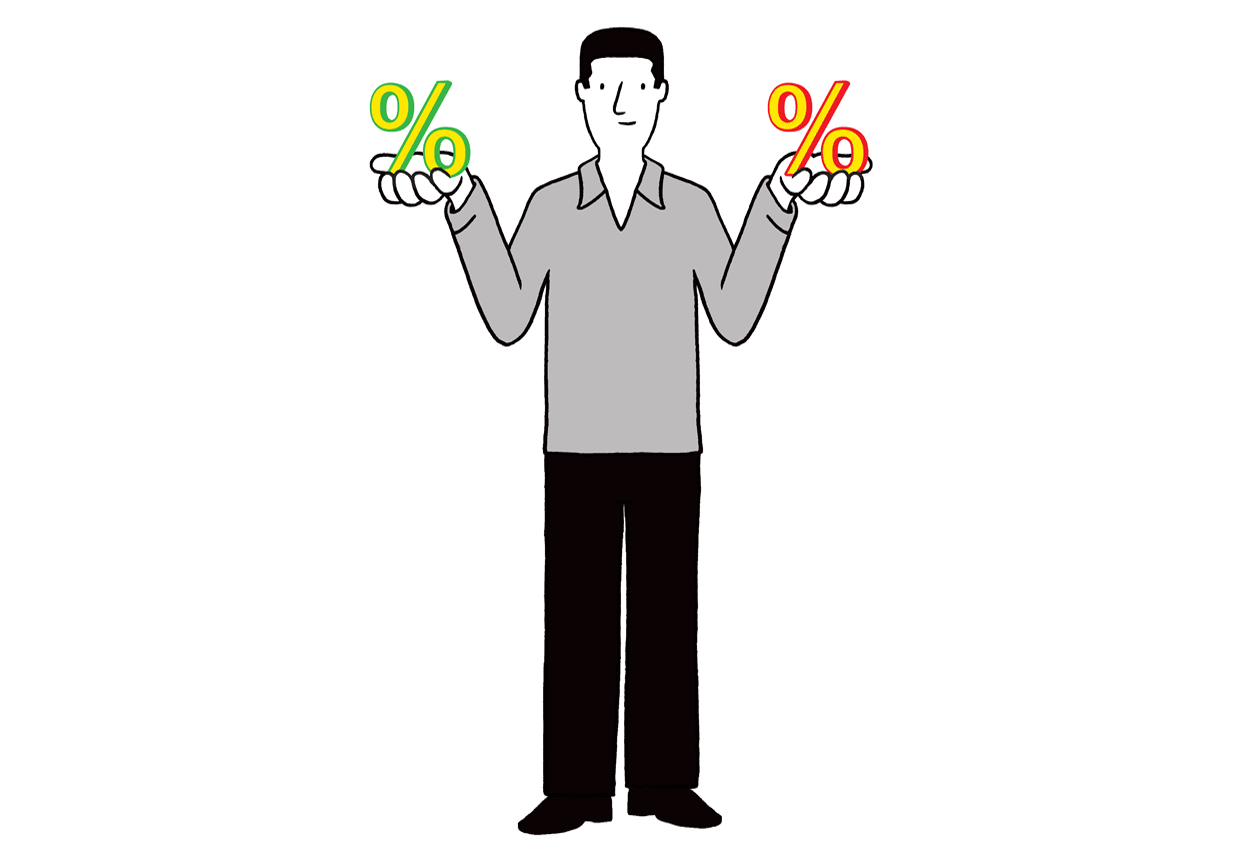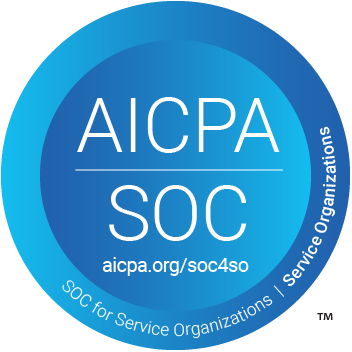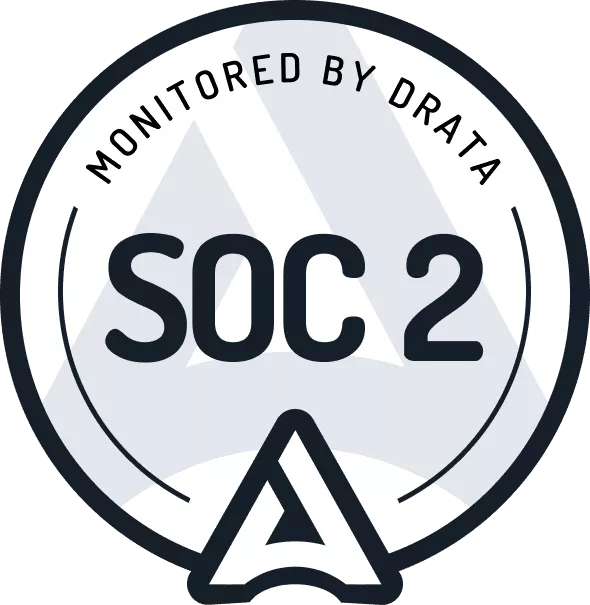# How to Calculate the Discount Rate for a Lease Under ASC 842

Lucas Russell 2021/03/31

When complying with ASC 842, the area that requires the most judgment is determining the discount rate that will be used to present value the future lease payments to calculate the lease liability.

The remaining inputs to calculate the lease liability are taken from the lease agreement itself, while the lessee must determine the discount rate. For capital leases under ASC 840, which are now referred to as finance leases under ASC 842, this is not a new concept.

For leases classified as operating leases under ASC 840, a discount rate was not required. Under ASC 842, given that all leases are now recorded on the balance sheet with a lease liability and right of use asset, the lessee must determine discount rates for operating leases as well.

This article will detail what the discount rate is under ASC 842 and how to upon what the most appropriate discount rate to use for a lease classified under ASC 842.

## What is the discount rate?

ASC 842 defines the discount rate as

For a lessee, the discount rate for the lease is the rate implicit in the lease unless that rate cannot be readily determined. In that case, the lessee is required to use its incremental borrowing rate.

The discount rate, as mentioned previously, is the cornerstone of the net present value calculation to calculate the lease liability.## How to calculate the discount rate

Based on the standard, the discount rate to apply is the rate implicit in the lease. If the lessee cannot calculate the rate implicit in the lease, the fallback is the incremental borrowing rate of the lease.

To learn how to calculate the rate implicit in the lease, refer to our article here How to Calculate the Implicit Rate in the Lease.

If, after reading the article, you cannot calculate the rate implicit in the lease, refer to our article on calculating the incremental borrowing rate of the lease How to Calculate the Incremental Borrowing Rate under ASC 842.

Also, if you want to use the most straightforward method, refer to this article How to Calculate the Discount Rate Using the Risk Free Rate.

## Can I use the same discount rate for all my agreements?

After reviewing the applicable guidance, it is apparent that this accounting judgment takes a significant determination of what the appropriate discount rate should be. So the next question is can a company use one discount rate for their lease portfolio.

This is only permitted when the lessee can conclude that the application of one discount rate does not create a material difference from individually determining discount rates applied to each of the leases in the portfolio. For this to occur, the leases should share the following characteristics:

• A similar value of lease payments
• Similar lease length

PwC provides an example of 20 leases in which the annual fixed payments range from \$3,750 to \$4,000, and the lease term for all leases is three years. PwC concluded that in this scenario, it would be acceptable to apply the same discount rate. The further the lease details diverge, the harder it to justify there is no material difference between not applying individual discount rates.

## Conclusion

Once the discount rate has been determined, you have all the pieces required to calculate the lease liability and the right of use asset. We have plenty of material to help with your lease accounting journey with:

## FooterHere at Cradle, our mission is simple; it's at the foundation of everything that we do. We want to make accountants' lives easier by leveraging technology to free up their time to focus on running the business.

140 Yonge St.
Suite 200
Toronto, ON M5C 1X6

US
Suite #73591
Torrance, CA 90503
USA

### Legal